# Turbulence and Energy Assessment of a Two Bladed H-Type Vertical Axis Wind Turbine Between Two High-Rise Buildings

Turbulence and Energy Assessment of a Two Bladed H-Type Vertical Axis Wind Turbine Between Two High-Rise Buildings
Faculty of Mechanical Engineering, Urmia University of Technology, Urmia, Iran

Faculty of Mechanical Engineering, Urmia University, Urmia, Iran

Corresponding Author Email:
sep.farid@yahoo.com
Page:
948-957
|
DOI:
https://doi.org/10.18280/ijht.370403
28 September 2019
|
Accepted:
17 November 2019
|
Published:
26 December 2019
| Citation

OPEN ACCESS

Abstract:

In the present study, the turbulence characteristics and efficiency of a two bladed H-type VAWT between two side-by-side high-rise buildings are evaluated numerically by means of fluid dynamics technique using the finite volume approach. The free-wind speeds of U=5 and 10 m/s, non-dimensional gap spaces of G/D=1.5 and 3.0, and tip speed ratios (TSR) of 3 and 5 are the parameters under consideration which are studied in detail. The obtained results for two side-by-side buildings in the absence of a VAWT and a VAWT without the buildings are validated against the available data in the open literature and found good agreements from both comparisons. Examination of obtained results reveals that, for gap spaces of G/D=1.5 and 3.0, 75% and 44%-45% augmentations in local wind speed on the center line between two high-rise buildings can be observed, respectively. The maximum improvements of VAWT efficiency are achieved as 21.8% and 15.1% for “G/D=1.5, U=10 m/s, and TSR=3” and “G/D=3.0, U=5 m/s, and TSR=5”, respectively.

Keywords:

vertical axis wind turbine (VAWT), high-rise buildings, wind potential, power coefficient

1. Introduction

By increasing global population, demand to use energy sources increases. Among these energy sources, fossil fuels are attractive because of their benefits to traders. Using fossil fuels can result in increasing greenhouse gases, global warming, pollution, acidic rain, polar ice caps melting, draining water resources, etc. The best solution to prevent the mentioned results of using fossil fuels, are renewable energy resources such as wind, solar, ocean waves, hydroelectric and geothermal. Wind energy can be harnessed in many places of the world at any time. It can be harnessed in residential and nonresidential places, coastlines, offshore, during the day and night and in many climate conditions. Wind turbines are used to capture wind energy source. They can be used individually in some residential places or with several turbines as a wind farm. Thus, designing parameters of wind turbines and layout of wind farms have significant importance in increasing efficiency of energy production. Nowadays, small-scale wind turbines have considerable roles in electricity generation not only in developed countries but also in developing ones. Two major types of wind turbines, which can be used in urban environments, are vertical axis wind turbine (VAWT) and horizontal axis wind turbine (HAWT). VAWTs are not sensitive to wind direction and wind turbulence and can be installed easily in comparison with HAWTs. Up to now, many different papers have been published about optimization of number of blades, blade profile and the developed wake flow downstream of the rotor. In the following, some previously published works are reviewed.

Several researchers have studied wake flow characteristics, turbulence effects, etc, in flow around a wind turbine. Rolland et al. [9, 10] investigated a VAWT experimentally and numerically. They studied the effects of rotor speed, wind speed, blade pitch angle and yaw angle on the power output of the turbine. Their results showed that by increasing the wind speed and yaw angle, the power output of the wind turbine was increased. Tescione et al.  studied turbulence parameters of flow behind a VAWT using the Particle Image Velocimetry (PIV) in an open-jet wind tunnel and measured streamwise and lateral velocity profiles at different locations downstream of the wind turbine. They showed that blade wakes were not detectable beyond x/R=3. Bausas and Danao  investigated the effect of free-wind turbulence on the performance of a camber-bladed VAWT (NACA 1425) and compared the results with symmetric bladed VAWT (NACA 0025). They showed that by increasing velocity fluctuations, power coefficient of camber-bladed wind turbine was decreased. Zuo et al.  simulated the effect of wake on the performance of a VAWT, numerically. They placed two wind turbines with 5D (diameter of rotor) to 17D horizontal distance and showed that by placing the second turbine at 12D distance from the first turbine, both turbines would have the same incoming flow characteristics. Chen et al.  simulated a system of two VAWTs and studied the effect of some parameters such as TSR, incoming flow angle, rotational direction, and turbine spacing and revealed that TSR and incoming flow angle have the most significant effect on the power coefficient of wind turbine. Cappanera et al.  carried out experimental and numerical investigation to study the effect of trailing-edge serrations on the noise emissions of wind turbine blades and concluded that trailing-edge serrations have not significant effect on the reduction of noise emissions of blades from fluid dynamics point of view.

The present study focuses on finding the proper locations to install a VAWT in residential and nonresidential places between two high-rise buildings in a side-by-side arrangement to achieve maximum performance. For this purpose, flow behavior between two high-rise buildings are computed numerically to find the appropriate points for locating a VAWT to achieve maximum performance. Then, a VAWT is located at these points to investigate its performance. Also, flow behavior around a single VAWT embedded in the free-wind flow is studied to compare the results with a VAWT between two high-rise buildings. Time-averaged velocity field, to indicate the wind potential map, and velocity fluctuation pattern, due to its impact on the turbine lifecycle, are two key parameters to make a decision for selecting the optimum locations.

2. Computational Domain

This study deals to a two bladed H-type Darrius VAWT performance assessment between two high rise buildings. In this way, in order to find the optimum locations of a VAWT installation between two high-rise buildings as a function of gap space and free-wind speed, flow around two side-by-side buildings in the absence of a VAWT is computed. Two different non-dimensional gap spaces of G/D=1.5 and 3.0 and free-wind speeds of U=5 and 10 m/s (corresponding to Re=5×106 and 107 based on the building width and free-wind speed) are studied. For this goal, a 2D flow domain as Figure 1 is defined. The 2D computations are performed based on this assumption that the installation height of the VAWT is far from the earth and buildings roofs. The high-rise buildings under consideration have the same dimensions with square cross-sections as demonstrated in Figure 1(a). The under consideration computational domain has dimensions of 31D and 21D+G in streamwise and lateral directions, respectively. The center of buildings is set at 10.5D from the incoming section and 10.5D from the nearest horizontal boundary. The dimensions of the computational domain for simulating two side-by-side buildings are large enough to avoid any boundary effect on the wake flow. Table 1 compares the dimensions of computational domain applied in the present study with previous works. Regarding the boundary conditions, uniform velocity, zero pressure, symmetry, and no-slip condition are adopted for the inlet section, outlet section, horizontal, and wall boundaries, respectively. In the second step, computations have been performed around a two bladed H-type Darrius VAWT in a free-wind flow for comparison and validation purposes. The 2D flow domain to investigate flow behavior around a VAWT under consideration is shown in Figure 1(b). The blades are constructed with NACA0018 airfoils which is used widely by researchers [6, 8, 11]. Table 2 presents more information of geometric specifications of VAWT and applied dimensions for the flow domain. Finally, in the last step, after finding the appropriate locations for installing a VAWT as a function of G/D and U from step one, a VAWT is embedded at specified points between two side-by-side buildings and its performance is evaluated and compared with conventional case (a VAWT in the absence of buildings).

## 1a.jpg(a) Two high-rise buildings

## 1b.jpg(b) VAWT

Figure 1. 2D flow domain

Table 1. 2D flow domain dimensions used by previous studies

 Authors VAWT simulation Square cylinder simulation L1 W1 L2 W2 Yang et al.  9Dt 3Dt - - Bausas and Danao  10Dt 10Dt - - Rezaeiha et al. [6-8] 20Dt 20Dt - - Octavianty and Asai  - - 6.5D 7D Hassanzadeh  - - 31D 21D Meghdir et al.  8D 3D Present study 30Dt 20Dt 31D 21D+G

Table 2. Geometrical specification of VAWT and 2D computational domain

 Parameter Value Airfoil NACA0018 Number of blades 2 Chord length 60 mm Shaft diameter (Ds) 40 mm Domain Length (L1) 30Dt Domain Width (W1) 20Dt Turbine diameter (Dt) 1000 mm Rotating zone diameter (Dr) 1500 mm
3. Governing Equations

In the present work, the incompressible turbulent flow around the wind turbine and two side-by-side high-rise buildings is assumed as transient and two-dimensional. Many researchers showed the accuracy of turbulent URANS equations which are used in this study to simulate turbulent effects. To investigate the flow behavior around a wind turbine, different types of turbulence models such as Transition SST and SST k-ω models are employed by several researchers such as [3, 4, 14]. According to available publications in the open literature, Transition SST turbulence model presents results very close to experimental data and therefore, this model is employed in this study. Consequently, the governing equations for 2D, unsteady, turbulent, and incompressible flow are given as follows;

Conservation of mass

$\nabla .\,\vec{V}=0$                    (1)

Conservation of momentum

$\rho \frac{D\vec{V}}{Dt}=-\nabla p+\mu {{\nabla }^{2}}\,\vec{V}$    (2)

The dimensionless coefficients are defined by the following equations :

${{C}_{D}}=\frac{{{F}_{D}}}{0.5\rho {{U}^{2}}S}$                  (3)

${{C}_{m}}=\frac{M}{0.5\rho {{U}^{2}}S}$               (4)

${{C}_{P}}=\frac{P}{0.5\rho {{U}^{3}}S}$                         (5)

$TSR=\frac{R\omega }{U}$                       (6)

Wind power potential is assessed as :

$\frac{P}{A}=\frac{1}{2}\rho {{V}^{3}}$                     (7)

Here, the governing equations are solved numerically by means of the finite volume method. For this goal, the advection and viscous terms are discretized using the second-order upwind and central differencing schemes, respectively. On the other hand, the time derivatives are discretized using a second-order implicit method. For velocity-pressure fields coupling, the SIMPLE algorithm is carried out. The convergence criteria for all flow variables are set to be less than 10-7.

4. Grid Size Independence Study

In this research, a comprehensive study has been carried out to ensure that the applied grids have sufficient resolutions with respect to the computational cost. In this regard, the grid size independence study is performed for flow around two high-rise buildings in the absence of a VAWT as well as the flow around a VAWT in the absence of buildings. For the simulation of flow between two high-rise buildings, grid study has been accomplished for U=10 m/s and G/D=3.0. Here, time-averaged total drag coefficient of buildings $\left( {{{\bar{C}}}_{{{D}_{total}}}}={{{\bar{C}}}_{{{D}_{building\,1}}}}+{{{\bar{C}}}_{{{D}_{building\,2}}}} \right)$ is the key parameter in this regard. Unstructured grids have been used throughout the domain, except near the wall of the buildings. In these regions, structured grids have been used to capture turbulence characteristics in viscous sublayer. Constructed grids for computational domain are shown in Figure 2. Instantaneous maximum y+ values on the wall of the buildings are shown in Table 3. It is clear that the maximum y+ value is less than 5. Seven different numbers of grids are used to study grid size independence. Details of the applied grids and obtained results are shown in Table 3. As illustrated in Table 3, after 338,000 control volumes, the deviations of obtained results converge to zero. Normalized time-averaged streamwise velocity profiles for selected grids at locations of x/D=2.5, 3.5 and 4.5, downstream of buildings, are shown in Figure 3. Examination of plots in Figure 3 reveals that, velocity profiles of grids number 5 and 6 are almost same.

## 2.jpg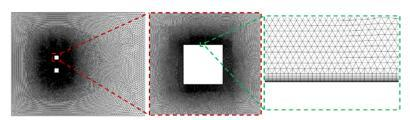Figure 2. Details of applied grids in this study for two side-by-side buildings

Table 3. Results of grid size independence study for two side-by-side high-rise buildings at G/D=3.0 and U=10 m/s

 Grid No. Number of cells ζmin/D y+max $\bar{C}_{D, \text {total}}$ Deviation (%) 1 57,000 4.167x10-4 4.13 2.74 -- 2 75,000 4.167x10-4 4.13 2.68 -2.19 3 112,000 4.167x10-4 4.13 2.55 -4.85 4 187,000 4.167x10-4 4.13 2.60 1.96 5 338,000 4.167x10-4 4.13 2.61 0.38 6 433,000 4.167x10-4 4.13 2.61 0 7 476,000 4.167x10-4 4.13 2.61 0

## 3.jpgFigure 3. Grid test results of normalized time-averaged streamwise velocity profiles at different locations downstream of buildings for U=10 m/s and G/D=3.0

On the other hand, grid test for the second case (VAWT in free-wind flow in the absence of buildings) has been performed for U=9.3 m/s and TSR=3.5 to be able to comparison with the experimental data of Tescione et al. . Here, power coefficient (CP) and momentum coefficient (Cm) are two key parameters in the second grid size independence study. Constructed grids for computational domain are shown in Figure 4. Like as the first case, unstructured grids have been used throughout the domain, except near wall of blades and the shaft. In these regions, structured grids have been used. Instantaneous maximum y+ value on the wall of blade for the last revolution of VAWT is depicted in Table 4. However, to obtain grid independent results, several grid resolutions have been examined according to the Table 4 using 70,000-759,000 control volumes. The obtained results of time-averaged power coefficient and instantaneous momentum coefficient in last revolution of the rotor are presented in Table 4. Here, after 375,000 control volumes, an ignorable variation has been observed with further grid refinement. This claim is confirmed by examination of the instantaneous momentum coefficient in the last revolution of the rotor which is presented for only “Grid 1”, “Grid 3”, and “Grid 5” in Figure 5. As a result, by taking into consideration of Figure 5 and Table 4, it is concluded that selecting “Grid 3” with 375,000 control volumes will lead to have results with high level of accuracy.

## 4.jpgFigure 4. Details of applied grids in this study for VAWT simulation

Table 4. Power coefficient and deviations for studied grids of VAWT simulation for U=9.3 m/s and TSR=3.5

 Grid No. Number of cells $\bar{C}_{P}$ Deviation (%) Maximum y+ on the blade Maximum y+ on the shaft 1 70,000 0.25 -- 2.86 0.456 2 192,000 0.28 12 2.86 0.456 3 375,000 0.29 0.04 2.86 0.456 4 522,000 0.29 0 2.86 0.456 5 759,000 0.29 0 2.86 0.456

## 5.pngFigure 5. Grid test results of momentum coefficient of rotor for selected grids at U=9.3 m/s and TSR=3.5

5. Time-Step Size Independence Study

In simulations of flow around two side-by-side buildings, various time-step sizes were used by several researchers [18, 22-26]. In this research, in addition to the grid size independence study, the time-step size independence study is carried out. The obtained results of time-step size independence study in terms of non-dimensional time-step of (dt*=dtxU/D) for simulation of two high-rise buildings under U=10 m/s and G/D=3.0 is illustrated in Figure 6. According to Figure 6, for the non-dimensional time-step size less than 0.0006, percentage of difference in total time-averaged drag coefficient is less than 0.72%. Furthermore, in Figure 7, normalized time-averaged streamwise velocity profiles downstream of buildings at x/D=2.5, 3.5 and 4.5 are plotted to evaluate time-step size independence study. According to Figure 7, normalized time-averaged streamwise velocity profiles of dt*=0.0006 and dt*=0.0003 are, more or less, equal. Thus, selecting a time-step size of dt*=0.0006 will ensure to have time-step independent results.

## 6.pngFigure 6. Time-step size independence study for simulation of two high-rise buildings at U=10 m/s and G/D=3.0

## 7.jpg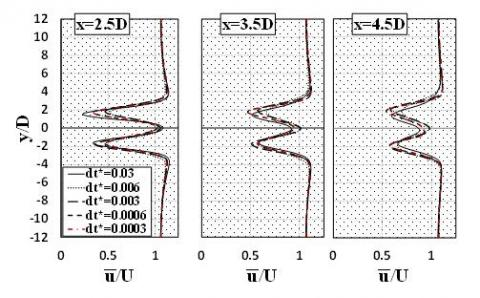Figure 7. Normalized time-averaged streamwise velocity profiles at different locations downstream of the buildings for time-step size independence study

On the other hand, in wind turbine simulations, among the researchers [6-8, 27-29], time-step size is chosen with respect to the azimuthal increment of the rotor. The azimuth angles between 0.1°-1° are popular in previously published works. Results of the time-step size study are shown in Figure 8 for a VAWT in the absence of the buildings. Instantaneous momentum coefficients of rotor for the last revolution of wind turbine is applied to evaluate the results. To compare the various time-step sizes, variations of the instantaneous momentum coefficients for last revolution at various time-step sizes such as 0.5°, 0.1°, and 0.05° of rotor azimuth angle have been examined. Examination of the results in Figure 8 reveals that momentum coefficients for time-step sizes based on 0.1° and 0.05° of rotor revolution have ignorable difference. Hence, 0.1° of revolution has been selected for time-step size of a VAWT. Comparison between the selected time-step sizes for the simulation of the building (without a VAWT) and a VAWT (without buildings) shows that the selected time-step size for a VAWT is smaller than that of the building case. Therefore, the time-step size of the VAWT is applied for the other main cases.

## 8.pngFigure 8. Instantaneous momentum coefficient in the last revolution of VAWT for time-step size independence study of VAWT simulation

6. Time Duration Independence Study

Time duration study of two high-rise buildings and VAWT simulations are shown in Figures 9 and 10, respectively. Total time-averaged drag coefficient and instantaneous momentum coefficient are used to study time duration of two high-rise buildings and VAWT, respectively. As shown in Figure 9, for simulation of two buildings, there is not a significant change in drag coefficient after non-dimensional time of 80 and total time-averaged drag coefficient of buildings remains almost constant. The percentage of differences in drag coefficients after non-dimensional time of t*=93.3, are less than 0.4%. For VAWT simulation, as illustrated in Figure 10, time duration is studied by examination of instantaneous momentum coefficient of VAWT for U=10 m/s and TSR=4.5. According to Figure 10, after t=1s (15 revolution of wind turbine), momentum coefficient of VAWT does not change significantly. Hence, in VAWT simulation, number of revolutions of wind turbine is applied more than 20 to ensure the accuracy of results. As a result, numerical simulation of VAWT should take at least 15 revolutions of rotor, and in simulation of two high-rise buildings non-dimensional time should be at least 80 to ensure the accuracy of the results.

## 9.pngFigure 9. Flow time duration study of simulation of two high-rise buildings for U=10 m/s and G/D=3.0

## 10.png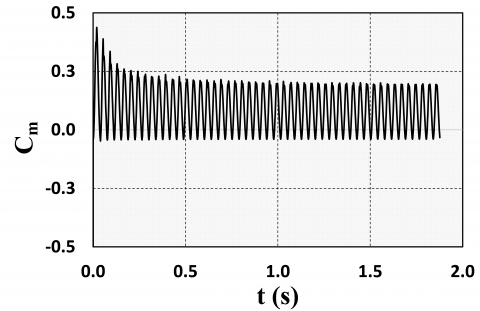Figure 10. Instantaneous momentum coefficient of VAWT for U=9.3 m/s and TSR=4.5

7. Validation

To validate numerical simulation of two high-rise buildings, experimental data of Alam et al.  and Yen and Liu  are used to show the accuracy of the numerical results. For this purpose, time-averaged total drag coefficient of buildings and normalized time-averaged streamwise and lateral velocities at the location of (x/D=2.5, y/D=0.0), according to experimental data, under G/D=1.5 and 3.0 at Reynolds numbers of Re=21,000 and Re=47,000 are applied. Table 5 shows these comparisons in detail. As demonstrated, the obtained numerical results in the present study for two side-by-side buildings in the absence of a VAWT are in good agreements with previous experimental data.

Table 5. Validation of present study with experimental works at (x/D=2.5, y/D=0.0) for simulation of two high-rise buildings as a function of Re and G/D

 Authors Re G/D $\bar{C}_{D}$ ͞u/U ͞v/U Alam et al.  47,000 1.5 1.50, 1.85 - - 3.0 2.11, 2.27 - - Yen and Liu  21,000 1.5 1.30, 1.38 0.63 -0.007 3.0 - 1.09 -0.016 Present study 47,000 1.5 1.83 - - 3.0 2.35 - - 21,000 1.5 1.48 0.72 -0.006 3.0 - 0.96 -0.014

Additionally, to validate numerical simulation of VAWT, experimental data of Tescione et al.  and numerical results of Rezaeiha et al.  are used to show the accuracy of present VAWT simulation. Normalized time-averaged streamwise velocity profiles are compared to evaluate the accuracy of the predicted results of the present study. Figure 11 compares time-averaged streamwise velocity profiles with experimental data of Tescione et al.  and numerical 2D and 2.5D results of Rezaeiha et al.  at x/R=2.5, 3.0 and 3.5 downstream of the VAWT which are normalized with respect to free-wind speed. In this comparison, good agreements are again observed between the present study and previous data.

## 11.jpg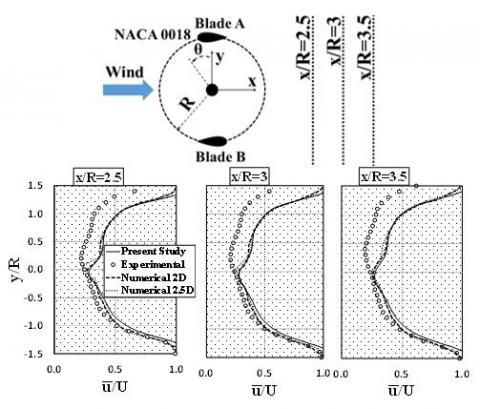Figure 11. Validation of the applied numerical code for the VAWT with experimental data of Tescione et al.  and numerical 2D and 2.5D results of Rezaeiha et al. ; comparisons of time-averaged streamwise velocity profiles

8. Results

Location of a VAWT between two high-rise buildings should be selected in a way to achieve more performance in comparison to the case without high-rise buildings. For this purpose, some key parameters such as distributions of velocity magnitudes between buildings should be considered to find the optimum points as a function of the Reynolds number and non-dimensional gap space. In this way, time-averaged normalized velocity profiles on the central line (y=0) between the buildings for G/D=1.5 and 3.0 under U=5 m/s and 10 m/s, are illustrated in Figure 12. As shown, with moving on the center line from the incoming section toward the outlet section, time-averaged normalized velocity magnitude attenuates gradually and falls to a minimum value in a specific position upstream of the buildings due to a high flow blockage. After this minimum position, a sudden jump in the velocity distributions can be observed which is occurred between the buildings due to development of a jet-like flow as stated before by several researchers. Exact locations of the maximum velocity on the center line and their magnitudes are shown in images of Figure 12. Further examination of the obtained results shows that by increasing the free-wind speed for G/D=3.0, location of maximum velocity between two buildings shifts toward the downstream side. By increasing free-wind speed from U=5 m/s to U=10 m/s, maximum enhancement of velocity between two buildings reaches to 75% for G/D=1.5, and 45% for G/D=3.0. Thus, it expects that by installing the VAWT at locations of x/D=-0.18 and x/D=-0.19 for the case of G/D=1.5 under U=5 m/s and 10 m/s, respectively, and at locations of x/D=0.28 and x/D=0.31 in the case of G/D=3.0 under U=5 m/s and 10 m/s, respectively, the energy efficient of the VAWT will enhance.

## 12.jpg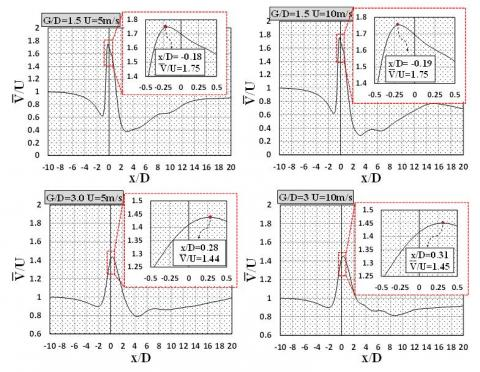Figure 12. Normalized time-averaged velocity profiles on the central line (y=0) between two high-rise buildings for different G/D and U values

Instantaneous momentum coefficient of the rotor for G/D=1.5 and 3.0 under U=10 m/s is depicted in Figure 13. For comparison purposes, the obtained corresponding results for rotor in the absence of the buildings are included in images of this figure. As it is shown in Figure 13, maximum momentum coefficient of rotor for “G/D=1.5, TSR=3” and “G/D=3.0, TSR=3” occurs at azimuth angles of 77° and 78°, respectively. Also, maximum momentum coefficient of rotor for “G/D=1.5, TSR=5” and “G/D=3.0, TSR=5” occurs at azimuthal angles of 102° and 95°, respectively. By increasing the TSR up to 5 at a constant non-dimensional gap space (G/D), maximum momentum coefficient occurs at higher azimuthal angles. In comparison to TSR=3, in the case of TSR=5 the maximum torque occurs at higher azimuth angles of rotor as shown in Figure 13.

Vorticity plots normalized by free-wind speed and rotor diameter have been shown at various azimuth angles for G/D=3.0 and U=5 m/s in figure 14. For enhanced visualization, the presented instantaneous contours are illustrated for TSR=3 and 5 at left and right-hand sides, respectively. High levels of vorticity can cause destructive effects in a wind turbine such as the vibration in blades, shaft, and the other parts due to fluctuations in velocity. Consequently, the lifecycle of the VAWT can be under the influence of this bad occurrence. According to Figure 14, regardless of the azimuth angle, at lower TSR such as 3, the developed vortical structures are considerable and occupy more regions within the rotor compared with TSR=5. This is due to this fact that by increasing the rotating speed of rotor, low volume of air can penetrate into the rotor and therefore, interaction between the air and rotor reduces. Thus, reducing regions with high vorticity inside the rotor causes increasing the performance of wind turbine.

## 13.jpgFigure 13. Instantaneous momentum coefficient of rotor for G/D=1.5 and 3.0 under U=10 m/s for the last revolution of rotor

## 14.jpgFigure 14. Non-dimensional instantaneous vorticity patterns for G/D=3.0 and U=5 m/s under TSR=3 and TSR=5 in different azimuthal angles

Time-averaged power coefficients of the VAWT are illustrated in Figure 15. Here, power coefficients of the VAWT between two buildings are compared with conventional cases (without buildings). Several comments can be stated for obtained results in Figure 15 as well as Table 6. First of all, time-averaged power coefficient of a VAWT between two side-by-side buildings is higher in comparison to the conventional case in the absence of buildings regardless of all parameters under consideration. Furthermore, the improvement of the rotor performance is more evident in a smaller gap space between two buildings of G/D=1.5 comparing to G/D=3.0 as illustrated in Figure 15 and Table 6. The power coefficient of the VAWT between two side-by-side high-rise buildings is higher in lower G/D values under TSR=3. But, in TSR=5, the larger gap spaces should be preferred to meet the higher rotor performance. In addition, with increasing the free-wind speed and TSR, the power coefficient of the VAWT enhances, regardless of G/D values. However, comparing to the conventional case, these augmentations in power coefficient are varied with TSR, G/D, and U. The maximum improvement in power coefficient of the two bladed H-type VAWT between two side-by-side high-rise buildings is achieved as 21.8% compared with conventional case at G/D=1.5, TSR=3, and U=10 m/s.

## 15.jpg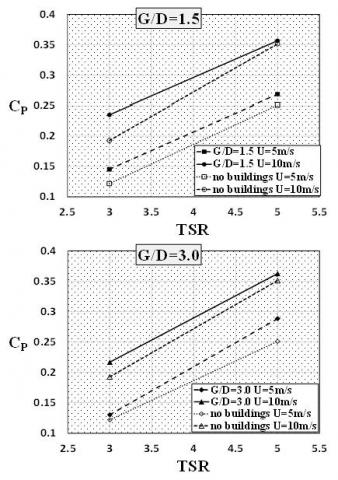Figure 15. Variations of time-averaged rotor Power coefficient with TSR, G/D, and U and comparisons with the corresponding conventional cases

Table 6. Power coefficient enhancement of the VAWT between two high-rise buildings compared with the case of no buildings (open space)

 G/D U (m/s) TSR Difference (%) 1.5 5 3 +18.9 5 +6.8 10 3 +21.8 5 +1.7 3.0 5 3 +6.6 5 +15.1 10 3 +12.4 5 +3.4
9. Conclusion

In this study, the aerodynamics performance of a two bladed H-type VAWT located between two side-by-side high-rise buildings has been evaluated two dimensionally. Two various non-dimensional gap spaces of G/D=1.5 and 3.0 along with TSR values of 3 and 5 were carried out under free-wind speeds of 5 and 10 m/s. In this way, firstly, the fluid dynamics around two side-by-side buildings was studied to find the optimum locations with high wind potential. It was found that for G/D=1.5, locations of x/D=-0.18 and -0.19 (both with 75% higher wind speed) and for G/D=3.0, locations of x/D=0.28 and 0.31 (both with 44%-45% higher wind speed) were found as four different optimum locations having the maximum wind potential on the center line between the side-by-side buildings. In the second step, the performance of a two bladed H-Type VAWT was investigated at the obtained optimum positions. Additionally, the VAWT in the absence of the buildings was also computed at corresponding TSR values for comparison purposes. Several quantitative and qualitative results have been presented in this numerical study. It was found that locating the VAWT at specified optimum points between two high-rise buildings augments the power characteristics of the rotor. Finally, it was revealed that by locating the VAWT under consideration at aforementioned optimum positions, the power characteristics of the rotor enhance with 1.7%-21.8% for G/D=1.5 and G/D=3.0, depending on the TSR and U values. However, the maximum improvement of 21.8% can be reported for G/D=1.5, U=10 m/s, and TSR=3. It was hoped that the obtained results arouse interest among the VAWT designers and installers.

Nomenclature
 A Area [m2] CD Drag coefficient [-] Cm Momentum coefficient [-] CP Power coefficient [-] D Width of building [m] Dr Diameter of rotating zone [m] Ds Diameter of shaft [m] Dt Diameter of turbine [m] dt* Non-dimensional time step [-] G Center-to-center gap space between buildings [m] M Torque [N.m] P Power [J/s] R Rotor radius [m] Re Reynolds number [-] S Rotor Swept Area [m2] t* Non-dimensional time [-] U Free-wind speed [m/s] V Local Velocity [m/s] $\vec{V}$ Velocity Vector y+ Dimensionless wall distance [-] Greek symbols $\mu$ Dynamic viscosity [kg/m.s] $\theta$ Azimuth angle [ o ] $\omega$ Angular velocity [rad/s] $\rho$ Density [kg/m3] ζ Element size Superscripts ͞ Time-averaged
References

 Li, Q.A., Maeda, T., Kamada, Y., Murata, J., Furukawa, K., Yamamoto, M. (2015). Effect of number of blades on aerodynamic forces on a straight-bladed vertical axis wind turbine. Energy, 90(Part 1): 784-795. https://doi.org/10.1016/j.energy.2015.07.115

 Li, Q.A., Maeda, T., Kamada, Y., Murata, J., Yamamoto, M., Ogasawara, T., Shimizu, K., Kogaki, T. (2016). Study on power performance for straight-bladed vertical axis wind turbine by field and wind tunnel test. Renewable Energy, 90: 291-300. https://doi.org/10.1016/j.renene.2016.01.002

 Bhargav, M.M.S.R.S., Ratna Kishore, V., Laxman, V. (2016). Influence of fluctuating wind conditions on vertical axis wind turbine using a three dimensional CFD model. Journal of Wind Engineering and Industrial Aerodynamics, 158: 98-108. https://doi.org/10.1016/j.jweia.2016.10.001

 Jafaryar, M., Kamrani, R., Gorji-Bandpy, M., Hatami, M., Ganji, D.D. (2016). Numerical optimization of the asymmetric blades mounted on a vertical axis cross-flow wind turbine. International Communications in Heat and Mass Transfer, 70: 93-104. https://doi.org/10.1016/j.icheatmasstransfer.2015.12.003

 Wang, Y., Sun, X., Dong, X., Zhu, B., Huang, D., Zheng, Z. (2016). Numerical investigation on aerodynamic performance of a novel vertical axis wind turbine with adaptive blades. Energy Conversion and Management, 108: 275-286. https://doi.org/10.1016/j.enconman.2015.11.003

 Rezaeiha, A., Kalkman, I., Blocken, B. (2017). CFD simulation of a vertical axis wind turbine operating at a moderate tip speed ratio: Guidelines for minimum domain size and azimuthal increment. Renewable Energy, 107: 373-385. https://doi.org/10.1016/j.renene.2017.02.006

 Rezaeiha, A., Kalkman, I., Blocken, B. (2017). Effect of pitch angle on power performance and aerodynamics of a vertical axis wind turbine, Applied Energy, 197: 132-150. https://doi.org/10.1016/j.apenergy.2017.03.128

 Rezaeiha, A., Montazeri, H., Blocken, B. (2018). Towards accurate CFD simulations of vertical axis wind turbines at different tip speed ratios and solidities: Guidelines for azimuthal increment, domain size and convergence. Energy Conversion and Management, 156: 301-316. https://doi.org/10.1016/j.enconman.2017.11.026

 Rolland, S., Newton, W., Williams, A.J., Croft, T.N., Gethin, D.T., Cross, M. (2013). Simulations technique for the design of a vertical axis wind turbine device with experimental validation. Applied Energy, 111: 1195-1203. https://doi.org/10.1016/j.apenergy.2013.04.026

 Rolland,  S.A., Thatcher, M., Newton, W., Williams, A.J., Croft, T.N., Gethin, D.T., Cross, M. (2013). Benchmark experiments for simulations of a vertical axis wind turbine. Applied Energy, 111: 1183-1194. https://doi.org/10.1016/j.apenergy.2013.06.042

 Tescione, G., Ragni, D., He, C., Simão Ferreira, C.J., van Bussel, G.J.W. (2014). Near wake flow analysis of a vertical axis wind turbine by stereoscopic particle image velocimetry. Renewable Energy, 70: 47-61. https://doi.org/10.1016/j.renene.2014.02.042

 Bausas, M.D., Danao, L.A.M. (2015). The aerodynamics of a camber-bladed vertical axis wind turbine in unsteady wind. Energy, 93(Part 1): 1155-1164. https://doi.org/10.1016/j.energy.2015.09.120

 Zuo, W., Wang, X., Kang, S. (2016). Numerical simulations on the wake effect of H-type vertical axis wind turbines. Energy, 106: 691-700. https://doi.org/10.1016/j.energy.2016.02.127

 Chen, W.H., Chen, C.Y., Huang, C.Y., Hwang, C.J. (2017). Power output analysis and optimization of two straight-bladed vertical-axis wind turbines. Applied Energy, 185(Part 1): 223-232. https://doi.org/10.1016/j.apenergy.2016.10.076

 Cappanera, G., D’Alessandro, V., Giammichele, L., Ricci, R. (2019). Acoustic investigation of aerodynamic appendages for wind turbine blades: Fluid-dynamic tests. TECNICA ITALIANA-Italian Journal of Engineering Science, 63(2-4): 329-335. https://doi.org/10.18280/ti-ijes.632-431

 Yang, M.H., Huang, G.M., Yeh, R.H. (2016). Performance investigation of an innovative vertical axis turbine consisting of deflectable blades. Applied Energy, 179: 875-887. https://doi.org/10.1016/j.apenergy.2016.07.072

 Octavianty, R., Asai, M. (2016). Effects of short splitter plates on vortex shedding and sound generation in flow past two side-by-side square cylinders. Experiments in Fluids, 57(9): 143. https://doi.org/10.1007/s00348-016-2227-4

 Hassanzadeh, R. (2017). Best location of the small wind turbine around a single high-rise building. International Journal of Green Energy, 14(2): 196-204. https://doi.org/10.1080/15435075.2016.1253581

 Meghdir, A., Benabdallah, T., Dellil, A.Z.E. (2019). Impact of geometry of electronic components on cooling improvement. International Journal of Heat and Technology, 37(1): 167-178. https://doi.org/10.18280/ijht.370121

 Hassanzadeh, R., Mohammadnejad, M. (2019). Effects of inward and outward overlap ratios on the two-blade Savonius type of vertical axis wind turbine performance. International Journal of Green Energy, 1-12. https://doi.org/10.1080/15435075.2019.1671420

 Jain, P. (2011). Wind Energy Engineering. New York: McGraw-Hill.

 Cao, Y., Tamura, T. (2017). Supercritical flows past a square cylinder with rounded corners. Physics of Fluids, 29(8): 085110. https://doi.org/10.1063/1.4998739

 Çuhadaroğlu, B., Turan, O. (2009). Numerical simulation of turbulent flow around a square cylinder with uniform injection or suction and heat transfer. Numerical Heat Transfer, Part A: Applications, 55(2): 163-184. https://doi.org/10.1080/10407780802603196

 Karabelas, S. (2010). Large eddy simulation of high-Reynolds number flow past a rotating cylinder. International Journal of Heat and Fluid Flow, 31(4): 518-527. https://doi.org/10.1016/j.ijheatfluidflow.2010.02.010

 Trias, F.X., Gorobets, A., Oliva, A. (2015). Turbulent flow around a square cylinder at Reynolds number 22,000: A DNS study. Computers & Fluids, 123: 87-98. https://doi.org/10.1016/j.compfluid.2015.09.013

 Xu, F.Y., Ying, X.Y., Zhang, Z. (2011). Prediction of unsteady flow around a square cylinder using RANS. Applied Mechanics and Materials, 52-54: 1165-1170. https://doi.org/10.4028/www.scientific.net/AMM.52-54.1165

 Balduzzi, F., Bianchini, A., Maleci, R., Ferrara, G., Ferrari, L. (2016). Critical issues in the CFD simulatin of Darrieus wind turbines. Renewable Energy, 85: 419-435. https://doi.org/10.1016/j.renene.2015.06.048

 Castelli, M.R., Ardizzon, G., Battisti, L., Benini, E., Pavesi, G. (2010). Modeling strategy and numerical validation for a Darrieus vertical axis micro-wind turbine. ASME 2010 International Mechanical Engineering Congress and Exposition, American Society of Mechanical Engineers, pp. 409-418. https://doi.org/10.1115/IMECE2010-39548

 Hashem, I., Mohamed, M.H. (2018). Aerodynamic performance enhancements of H-rotor Darrieus wind turbine. Energy, 142: 531-545. https://doi.org/10.1016/j.energy.2017.10.036

 Alam, M.M., Zhou, Y., Wang, X. (2011). The wake of two side-by-side square cylinders. Journal of Fluid Mechanics, 669: 432-471. https://doi.org/10.1017/S0022112010005288

 Yen, S.C., Liu, J.H. (2011). Wake flow behind two side-by-side square cylinders. International Journal of Heat and Fluid Flow, 32(1): 41-51. https://doi.org/10.1016/j.ijheatfluidflow.2010.09.005